Solves all kinds of geographical position calculations.

## nvector

The nvector library is a suite of tools written in Python to solve geographical position calculations. Currently the following operations are implemented:

• Calculate the surface distance between two geographical positions.

• Convert positions given in one reference frame into another reference frame.

• Find the destination point given start point, azimuth/bearing and distance.

• Find the mean position (center/midpoint) of several geographical positions.

• Find the intersection between two paths.

• Find the cross track distance between a path and a position.

Using n-vector, the calculations become simple and non-singular. Full accuracy is achieved for any global position (and for any distance).

### Description

In this library, we represent position with an “n-vector”, which is the normal vector to the Earth model (the same reference ellipsoid that is used for latitude and longitude). When using n-vector, all Earth-positions are treated equally, and there is no need to worry about singularities or discontinuities. An additional benefit with using n-vector is that many position calculations can be solved with simple vector algebra (e.g. dot product and cross product).

Converting between n-vector and latitude/longitude is unambiguous and easy using the provided functions.

n_E is n-vector in the program code, while in documents we use nE. E denotes an Earth-fixed coordinate frame, and it indicates that the three components of n-vector are along the three axes of E. More details about the notation and reference frames can be found in the documentation.

### Documentation and code

Official documentation:

http://www.navlab.net/nvector/

A Nonsingular Horizontal Position Representation, The Journal of Navigation, Volume 63, Issue 03, pp 395-417, July 2010.

Bleeding edge: https://github.com/pbrod/nvector.

Official releases available at: http://pypi.python.org/pypi/nvector.

### Install nvector

If you have pip installed and are online, then simply type:

$pip install nvector to get the lastest stable version. Using pip also has the advantage that all requirements are automatically installed. You can download nvector and all dependencies to a folder “pkg”, by the following:$ pip install –download=pkg nvector

$pip install –no-index –find-links=pkg nvector ### Verifying installation To verify that nvector can be seen by Python, type python from your shell. Then at the Python prompt, try to import nvector: >>> import nvector as nv >>> print(nv.__version__) 0.7.7dev0 To test if the toolbox is working correctly paste the following in an interactive python session: import nvector as nv nv.test('--doctest-modules') or$ py.test –pyargs nvector –doctest-modules

at the command prompt.

### Getting Started

Below the object-oriented solution to some common geodesic problems are given. In the first example the functional solution is also given. The functional solutions to the remaining problems can be found in the functional examples section of the tutorial.

#### Example 1: “A and B to delta”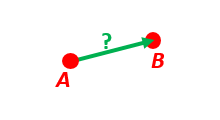Given two positions, A and B as latitudes, longitudes and depths relative to Earth, E.

Find the exact vector between the two positions, given in meters north, east, and down, and find the direction (azimuth) to B, relative to north. Assume WGS-84 ellipsoid. The given depths are from the ellipsoid surface. Use position A to define north, east, and down directions. (Due to the curvature of Earth and different directions to the North Pole, the north, east, and down directions will change (relative to Earth) for different places. Position A must be outside the poles for the north and east directions to be defined.)

Solution:
>>> import numpy as np
>>> import nvector as nv
>>> wgs84 = nv.FrameE(name='WGS84')
>>> pointA = wgs84.GeoPoint(latitude=1, longitude=2, z=3, degrees=True)
>>> pointB = wgs84.GeoPoint(latitude=4, longitude=5, z=6, degrees=True)

Step1: Find p_AB_N (delta decomposed in N).
>>> p_AB_N = pointA.delta_to(pointB)
>>> x, y, z = p_AB_N.pvector.ravel()
>>> 'Ex1: delta north, east, down = {0:8.2f}, {1:8.2f}, {2:8.2f}'.format(x, y, z)
'Ex1: delta north, east, down = 331730.23, 332997.87, 17404.27'

Step2: Also find the direction (azimuth) to B, relative to north:
>>> 'azimuth = {0:4.2f} deg'.format(p_AB_N.azimuth_deg)
'azimuth = 45.11 deg'
>>> 'elevation = {0:4.2f} deg'.format(p_AB_N.elevation_deg)
'elevation = 2.12 deg'
>>> 'distance = {0:4.2f} m'.format(p_AB_N.length)
'distance = 470356.72 m'

Functional Solution:
>>> import numpy as np
>>> import nvector as nv
>>> from nvector import rad, deg

>>> lat_EA, lon_EA, z_EA = rad(1), rad(2), 3

Step1: Convert to n-vectors:
>>> n_EA_E = nv.lat_lon2n_E(lat_EA, lon_EA)
>>> n_EB_E = nv.lat_lon2n_E(lat_EB, lon_EB)

Step2: Find p_AB_E (delta decomposed in E).WGS-84 ellipsoid is default:
>>> p_AB_E = nv.n_EA_E_and_n_EB_E2p_AB_E(n_EA_E, n_EB_E, z_EA, z_EB)

Step3: Find R_EN for position A:
>>> R_EN = nv.n_E2R_EN(n_EA_E)

Step4: Find p_AB_N (delta decomposed in N).
>>> p_AB_N = np.dot(R_EN.T, p_AB_E).ravel()
>>> x, y, z = p_AB_N
>>> 'Ex1: delta north, east, down = {0:8.2f}, {1:8.2f}, {2:8.2f}'.format(x, y, z)
'Ex1: delta north, east, down = 331730.23, 332997.87, 17404.27'

Step5: Also find the direction (azimuth) to B, relative to north:
>>> azimuth = np.arctan2(y, x)
>>> 'azimuth = {0:4.2f} deg'.format(deg(azimuth))
'azimuth = 45.11 deg'

>>> distance = np.linalg.norm(p_AB_N)
>>> elevation = np.arcsin(z / distance)
>>> 'elevation = {0:4.2f} deg'.format(deg(elevation))
'elevation = 2.12 deg'

>>> 'distance = {0:4.2f} m'.format(distance)
'distance = 470356.72 m'


Example 1 at www.navlab.net

#### Example 2: “B and delta to C”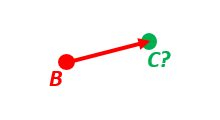A radar or sonar attached to a vehicle B (Body coordinate frame) measures the distance and direction to an object C. We assume that the distance and two angles (typically bearing and elevation relative to B) are already combined to the vector p_BC_B (i.e. the vector from B to C, decomposed in B). The position of B is given as n_EB_E and z_EB, and the orientation (attitude) of B is given as R_NB (this rotation matrix can be found from roll/pitch/yaw by using zyx2R).

Find the exact position of object C as n-vector and depth ( n_EC_E and z_EC ), assuming Earth ellipsoid with semi-major axis a and flattening f. For WGS-72, use a = 6 378 135 m and f = 1/298.26.

Solution:
>>> import numpy as np
>>> import nvector as nv
>>> wgs72 = nv.FrameE(name='WGS72')
>>> wgs72 = nv.FrameE(a=6378135, f=1.0/298.26)

Step 1: Position and orientation of B is given 400m above E:
>>> n_EB_E = wgs72.Nvector(nv.unit([, , ]), z=-400)
>>> frame_B = nv.FrameB(n_EB_E, yaw=10, pitch=20, roll=30, degrees=True)

Step 2: Delta BC decomposed in B
>>> p_BC_B = frame_B.Pvector(np.r_[3000, 2000, 100].reshape((-1, 1)))

Step 3: Decompose delta BC in E
>>> p_BC_E = p_BC_B.to_ecef_vector()

Step 4: Find point C by adding delta BC to EB
>>> p_EB_E = n_EB_E.to_ecef_vector()
>>> p_EC_E = p_EB_E + p_BC_E
>>> pointC = p_EC_E.to_geo_point()

>>> lat, lon, z = pointC.latlon_deg
>>> msg = 'Ex2: PosC: lat, lon = {:4.4f}, {:4.4f} deg,  height = {:4.2f} m'
>>> msg.format(lat, lon, -z)
'Ex2: PosC: lat, lon = 53.3264, 63.4681 deg,  height = 406.01 m'


Example 2 at www.navlab.net

#### Example 3: “ECEF-vector to geodetic latitude”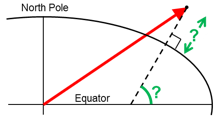Position B is given as an “ECEF-vector” p_EB_E (i.e. a vector from E, the center of the Earth, to B, decomposed in E). Find the geodetic latitude, longitude and height (latEB, lonEB and hEB), assuming WGS-84 ellipsoid.

Solution:
>>> import numpy as np
>>> import nvector as nv
>>> wgs84 = nv.FrameE(name='WGS84')
>>> position_B = 6371e3 * np.vstack((0.9, -1, 1.1))  # m
>>> p_EB_E = wgs84.ECEFvector(position_B)
>>> pointB = p_EB_E.to_geo_point()

>>> lat, lon, z = pointB.latlon_deg
>>> 'Ex3: Pos B: lat, lon = {:4.4f}, {:4.4f} deg, height = {:9.3f} m'.format(lat, lon, -z)
'Ex3: Pos B: lat, lon = 39.3787, -48.0128 deg, height = 4702059.834 m'


Example 3 at www.navlab.net

#### Example 4: “Geodetic latitude to ECEF-vector”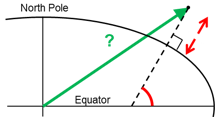Geodetic latitude, longitude and height are given for position B as latEB, lonEB and hEB, find the ECEF-vector for this position, p_EB_E.

Solution:
>>> import nvector as nv
>>> wgs84 = nv.FrameE(name='WGS84')
>>> pointB = wgs84.GeoPoint(latitude=1, longitude=2, z=-3, degrees=True)
>>> p_EB_E = pointB.to_ecef_vector()

>>> 'Ex4: p_EB_E = {} m'.format(p_EB_E.pvector.ravel().tolist())
'Ex4: p_EB_E = [6373290.277218279, 222560.20067473652, 110568.82718178593] m'


Example 4 at www.navlab.net

#### Example 5: “Surface distance”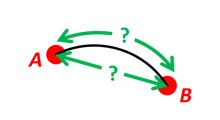Find the surface distance sAB (i.e. great circle distance) between two positions A and B. The heights of A and B are ignored, i.e. if they don’t have zero height, we seek the distance between the points that are at the surface of the Earth, directly above/below A and B. The Euclidean distance (chord length) dAB should also be found. Use Earth radius 6371e3 m. Compare the results with exact calculations for the WGS-84 ellipsoid.

Solution for a sphere:
>>> import numpy as np
>>> import nvector as nv
>>> frame_E = nv.FrameE(a=6371e3, f=0)
>>> positionA = frame_E.GeoPoint(latitude=88, longitude=0, degrees=True)
>>> positionB = frame_E.GeoPoint(latitude=89, longitude=-170, degrees=True)

>>> s_AB, azia, azib = positionA.distance_and_azimuth(positionB)
>>> p_AB_E = positionB.to_ecef_vector() - positionA.to_ecef_vector()
>>> d_AB = p_AB_E.length

>>> msg = 'Ex5: Great circle and Euclidean distance = {}'
>>> msg = msg.format('{:5.2f} km, {:5.2f} km')
>>> msg.format(s_AB / 1000, d_AB / 1000)
'Ex5: Great circle and Euclidean distance = 332.46 km, 332.42 km'

Alternative sphere solution:
>>> path = nv.GeoPath(positionA, positionB)
>>> s_AB2 = path.track_distance(method='greatcircle')
>>> d_AB2 = path.track_distance(method='euclidean')
>>> msg.format(s_AB2 / 1000, d_AB2 / 1000)
'Ex5: Great circle and Euclidean distance = 332.46 km, 332.42 km'

Exact solution for the WGS84 ellipsoid:
>>> wgs84 = nv.FrameE(name='WGS84')
>>> point1 = wgs84.GeoPoint(latitude=88, longitude=0, degrees=True)
>>> point2 = wgs84.GeoPoint(latitude=89, longitude=-170, degrees=True)
>>> s_12, azi1, azi2 = point1.distance_and_azimuth(point2)

>>> p_12_E = point2.to_ecef_vector() - point1.to_ecef_vector()
>>> d_12 = p_12_E.length
>>> msg = 'Ellipsoidal and Euclidean distance = {:5.2f} km, {:5.2f} km'
>>> msg.format(s_12 / 1000, d_12 / 1000)
'Ellipsoidal and Euclidean distance = 333.95 km, 333.91 km'


Example 5 at www.navlab.net

#### Example 6 “Interpolated position”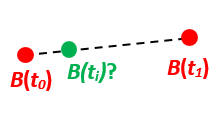Given the position of B at time t0 and t1, n_EB_E(t0) and n_EB_E(t1).

Find an interpolated position at time ti, n_EB_E(ti). All positions are given as n-vectors.

Solution:
>>> import nvector as nv
>>> wgs84 = nv.FrameE(name='WGS84')
>>> n_EB_E_t0 = wgs84.GeoPoint(89, 0, degrees=True).to_nvector()
>>> n_EB_E_t1 = wgs84.GeoPoint(89, 180, degrees=True).to_nvector()
>>> path = nv.GeoPath(n_EB_E_t0, n_EB_E_t1)

>>> t0 = 10.
>>> t1 = 20.
>>> ti = 16.  # time of interpolation
>>> ti_n = (ti - t0) / (t1 - t0) # normalized time of interpolation

>>> g_EB_E_ti = path.interpolate(ti_n).to_geo_point()

>>> lat_ti, lon_ti, z_ti = g_EB_E_ti.latlon_deg
>>> msg = 'Ex6, Interpolated position: lat, lon = {:2.1f} deg, {:2.1f} deg'
>>> msg.format(lat_ti, lon_ti)
'Ex6, Interpolated position: lat, lon = 89.8 deg, 180.0 deg'

Vectorized solution:
>>> t = np.array([10, 20])
>>> nvectors = wgs84.GeoPoint([89, 89], [0, 180], degrees=True).to_nvector()
>>> nvectors_i = nvectors.interpolate(ti, t, kind='linear')
>>> lati, loni, zi = nvectors_i.to_geo_point().latlon_deg
>>> msg.format(lat_ti, lon_ti)
'Ex6, Interpolated position: lat, lon = 89.8 deg, 180.0 deg'


Example 6 at www.navlab.net

#### Example 7: “Mean position”Three positions A, B, and C are given as n-vectors n_EA_E, n_EB_E, and n_EC_E. Find the mean position, M, given as n_EM_E. Note that the calculation is independent of the depths of the positions.

Solution:
>>> import nvector as nv
>>> points = nv.GeoPoint(latitude=[90, 60, 50],
...                      longitude=[0, 10, -20], degrees=True)
>>> nvectors = points.to_nvector()
>>> n_EM_E = nvectors.mean()
>>> g_EM_E = n_EM_E.to_geo_point()
>>> lat, lon = g_EM_E.latitude_deg, g_EM_E.longitude_deg
>>> msg = 'Ex7: Pos M: lat, lon = {:4.4f}, {:4.4f} deg'
>>> msg.format(lat, lon)
'Ex7: Pos M: lat, lon = 67.2362, -6.9175 deg'


Example 7 at www.navlab.net

#### Example 8: “A and azimuth/distance to B”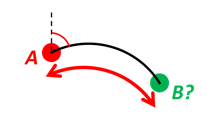We have an initial position A, direction of travel given as an azimuth (bearing) relative to north (clockwise), and finally the distance to travel along a great circle given as sAB. Use Earth radius 6371e3 m to find the destination point B.

In geodesy this is known as “The first geodetic problem” or “The direct geodetic problem” for a sphere, and we see that this is similar to Example 2, but now the delta is given as an azimuth and a great circle distance. (“The second/inverse geodetic problem” for a sphere is already solved in Examples 1 and 5.)

Exact solution:
>>> import numpy as np
>>> import nvector as nv
>>> frame = nv.FrameE(a=6371e3, f=0)
>>> pointA = frame.GeoPoint(latitude=80, longitude=-90, degrees=True)
>>> pointB, azimuthb = pointA.displace(distance=1000, azimuth=200, degrees=True)
>>> lat, lon = pointB.latitude_deg, pointB.longitude_deg

>>> msg = 'Ex8, Destination: lat, lon = {:4.4f} deg, {:4.4f} deg'
>>> msg.format(lat, lon)
'Ex8, Destination: lat, lon = 79.9915 deg, -90.0177 deg'

>>> np.allclose(azimuthb, -160.01742926820506)
True

Greatcircle solution:
>>> pointB2, azimuthb = pointA.displace(distance=1000,
...                                     azimuth=200,
...                                     degrees=True,
...                                     method='greatcircle')
>>> lat2, lon2 = pointB2.latitude_deg, pointB.longitude_deg
>>> msg.format(lat2, lon2)
'Ex8, Destination: lat, lon = 79.9915 deg, -90.0177 deg'

>>> np.allclose(azimuthb, -160.0174292682187)
True


Example 8 at www.navlab.net

#### Example 9: “Intersection of two paths”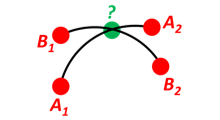Define a path from two given positions (at the surface of a spherical Earth), as the great circle that goes through the two points.

Path A is given by A1 and A2, while path B is given by B1 and B2.

Find the position C where the two great circles intersect.

Solution:
>>> import nvector as nv
>>> pointA1 = nv.GeoPoint(10, 20, degrees=True)
>>> pointA2 = nv.GeoPoint(30, 40, degrees=True)
>>> pointB1 = nv.GeoPoint(50, 60, degrees=True)
>>> pointB2 = nv.GeoPoint(70, 80, degrees=True)
>>> pathA = nv.GeoPath(pointA1, pointA2)
>>> pathB = nv.GeoPath(pointB1, pointB2)

>>> pointC = pathA.intersect(pathB)
>>> pointC = pointC.to_geo_point()
>>> lat, lon = pointC.latitude_deg, pointC.longitude_deg
>>> msg = 'Ex9, Intersection: lat, lon = {:4.4f}, {:4.4f} deg'
>>> msg.format(lat, lon)
'Ex9, Intersection: lat, lon = 40.3186, 55.9019 deg'

Check that PointC is not between A1 and A2 or B1 and B2:
>>> pathA.on_path(pointC)
False
>>> pathB.on_path(pointC)
False

Check that PointC is on the great circle going through path A and path B:
>>> pathA.on_great_circle(pointC)
True
>>> pathB.on_great_circle(pointC)
True


Example 9 at www.navlab.net

#### Example 10: “Cross track distance”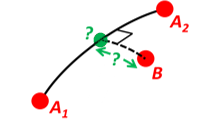Path A is given by the two positions A1 and A2 (similar to the previous example).

Find the cross track distance sxt between the path A (i.e. the great circle through A1 and A2) and the position B (i.e. the shortest distance at the surface, between the great circle and B).

Also find the Euclidean distance dxt between B and the plane defined by the great circle. Use Earth radius 6371e3.

Finally, find the intersection point on the great circle and determine if it is between position A1 and A2.

Solution:
>>> import numpy as np
>>> import nvector as nv
>>> frame = nv.FrameE(a=6371e3, f=0)
>>> pointA1 = frame.GeoPoint(0, 0, degrees=True)
>>> pointA2 = frame.GeoPoint(10, 0, degrees=True)
>>> pointB = frame.GeoPoint(1, 0.1, degrees=True)
>>> pathA = nv.GeoPath(pointA1, pointA2)

>>> s_xt = pathA.cross_track_distance(pointB, method='greatcircle')
>>> d_xt = pathA.cross_track_distance(pointB, method='euclidean')

>>> val_txt = '{:4.2f} km, {:4.2f} km'.format(s_xt/1000, d_xt/1000)
>>> 'Ex10: Cross track distance: s_xt, d_xt = {}'.format(val_txt)
'Ex10: Cross track distance: s_xt, d_xt = 11.12 km, 11.12 km'

>>> pointC = pathA.closest_point_on_great_circle(pointB)
>>> np.allclose(pathA.on_path(pointC), True)
True


Example 10 at www.navlab.net

### Acknowledgements

The nvector package for Python was written by Per A. Brodtkorb at FFI (The Norwegian Defence Research Establishment) based on the nvector toolbox for Matlab written by the navigation group at FFI. The nvector.core and nvector.rotation module is a vectorized reimplementation of the matlab nvector toolbox while the nvector.objects module is a new easy to use object oriented user interface to the nvector core functionality documented in [GB20].

Most of the content is based on the article by K. Gade [Gad10].

However, if you use any of the FrameE.direct, FrameE.inverse, GeoPoint.distance_and_azimuth or GeoPoint.displace methods you should also cite the article by Karney [Kar13] because these methods call Karney’s geographiclib library to do the calculations.

## Project details

This version0.7.70.7.60.7.50.7.40.7.30.7.00.6.00.5.20.4.10.1.3

Uploaded source
Uploaded py2 py3